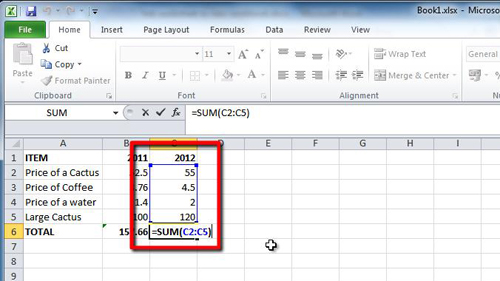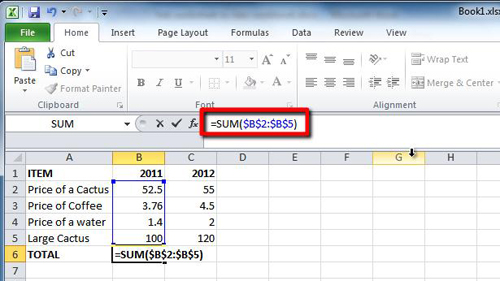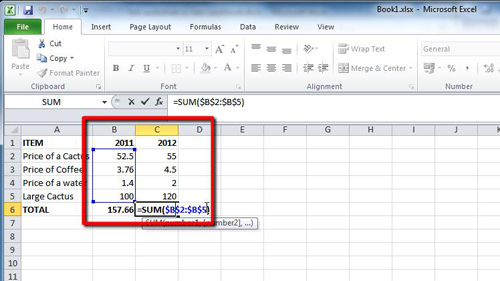## How to Make Excel 2010 formulas absolute

The default setting for formulas in excel is for them to be relative to the other data on the spreadsheet, this tutorial will show you how to make excel formulas absolute instead.

Step # 1 – Normal Relative Values

Normally in Excel if you copy the cell with a formula in such as the SUM formula in cell “B6” when you paste it in a new cell it will also change where it is getting its data from. Here we can see that the formula in “B6” takes the information from cell “B2” to “B5”. The new formula however takes the information from “C2” to “C5”. While this is a useful feature it is not always required.Step # 2 – Making a Formula Absolute

Let’s go back a step and make the formula absolute before we copy it. Delete the new formula we just pasted into cell “C5” and go back to cell “B6”, double click on it to edit the formula. To make it absolute you need to insert the “\$” symbol into the cell references. The formula should instead read “=SUM(\$B\$2:\$B\$5), then press return. This will mean that wherever the formula is moved to on the sheet it will still read from the range “B2” to “B5”.Step # 3 – Testing the Change

Now when we copy the same formula and paste it into cell “C6” it won’t change its value as it is still summing the data from the “2011” column. We can see this by double clicking on it and that is how to make excel formulas absolute.